This is an archive of the old MediaWiki-based ImageJ wiki. The current website can be found at imagej.net.

# Shape Filter

Shape Filter Plugin (ImageJ/Fiji)
Author Thorsten Wagner
Maintainer Thorsten Wagner
File shape_filter_x.y.z.jar 
Source Github 
Development status active

# General Description

The ImageJ Shape Filter Plugin use the [ij-blob] library to characterize and filter objects in binary scenes by its shape. Therefore, several features are calculated as shown below.

If you like to cite the Shape Filter plugin in a scientific publication, please cite:

Wagner, T and Lipinski, H 2013. IJBlob: An ImageJ Library for Connected Component Analysis and Shape Analysis. Journal of Open Research Software 1(1):e6, DOI: http://dx.doi.org/10.5334/jors.ae

## Shape Features

• Area (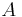$A$): The area enclosed by the outer contour of an object.
• Area Convex Hull (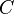$C$): The area enclosed by the convex hull of the outer contour of an object.
• Perimeter (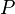$P$): The perimeter of the outer contour of an object.
• Perimeter Convex Hull (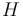$H$): The perimeter of the convex hull of the particle.
• Feret Diameter: The maximum distance between the two parallel tangents touching the particle outline in all directions.
• Min. Feret Diameter: the minimum distance between the two parallel tangents touching the particle outline in all directions.
• Max. Inscr. Circle Diameter: The diameter of the maximum inscribed circle.
• Area eq. circle diameter: Equivalent circle diameter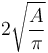$2\sqrt{\frac{A}{\pi}}$
• Long Side Minimum Bounding Rectangle (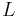$L$): The larger side of the minimum bounding rectangle.
• Short Side Minimum Bounding Rectangle (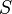$S$): The smaller side of the minimum bounding rectangle.
• Aspect Ratio: Defined as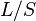$L/S$
• Area to Perimeter Ratio: Defined as$A/P$
• Circularity: Defined as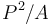$P^{2}/A$
• Elongation: Defined as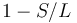$1 - S/L$
• Convexity: Defined as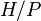$H/P$
• Solidity: Defined as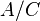$A/C$
• Number of Holes: The number of holes inside an object.
• Thinnes Ratio: Inverse proportional to the circularity. Furthermore it normed. It is defined as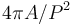$4\pi A/P^{2}$
• Contour Temperatur: It has a strong relationship to the fractal dimension, defined as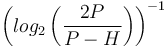$\left(log_{2}\left(\frac{2P}{P-H}\right)\right)^{-1}$
• Orientation: The orientation of the major axis from in grad (measured counter clockwise from the positive x axis).
• Fractal Box Dimension: Estimated fractal dimension by the box count algorithm. The default box-sizes are “2,3,4,6,8,12,16,32,64”.

# Installation

You could simply use our update site "Biomedgroup" to install the shape filter plugin.